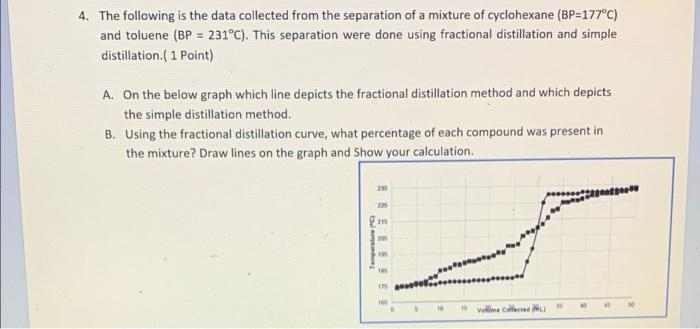# Question 4. The following is the data collected from the separation of a mixture of cyclohexane (BP=177°C) and toluene (BP = 231°C). This separation were done using fractional distillation and simple distillation.(1 Point) A. On the below graph which line depicts the fractional distillation method and which depicts the simple distillation method. B. Using the fractional distillation curve, what percentage of each compound was present in the mixture? Draw lines on the graph and Show your calculation 23 peate { 20 Temperatur Velme case